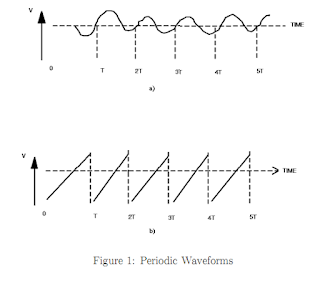## Posts

Showing posts from June, 2015

### The Cathode Ray OscilloscopeIntroduction  The following should give the student some familiarisation with the function and uses of the cathode ray oscilloscope (C.R.O.).

Consider a simple sine wave electrical signal from some source as in Fig. 1a. If we can arrange things so that this sinusoidal voltage is applied to two horizontal conducting plates then in the region between these plates, the electric field will be alternating with period T seconds. It will increase in strength to a maximum, decrease to zero, turn over, and increase in the opposite direction to an equal maximum, then decrease to zero again, in each period of time T.

Now, if there is a beam of charged particles (electrons) streaming between these horizontal plates, the oscillating electric field there will bend the beam first up, then down, then back to the undeflected position in each time period T. Further, if the beam strikes a plate of material which fluoresces, one would see a spot of light on this plate (screen) which moves vertically up …